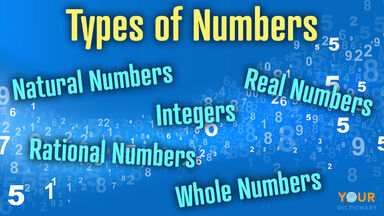# What Are the Different Types of Numbers in Math?

What is a number? The answer to that question might seem obvious, but there are actually different types of numbers. Expand your mathematical horizons by learning about the various kinds of numbers.types of numbers integers rational natural real whole

## Numerals

Any figure or symbol that is used to indicate a number is a numeral. Every way a number can be written, whether you're writing a small number or a large number, is an example of a numeral. This is true whether you are using standard Arabic numerals (0-9) in any combination or if you are using Roman numerals.

## Digits

Any Arabic numeral (0-9) is a digit. Digits are used to form natural numbers and whole numbers. When a natural number or a whole number is 9 or lower, it is also a digit. Natural or whole numbers higher than nine are formed by combining multiple digits.

## Natural Numbers

Natural numbers are the most basic type of number. They are generally the first numbers that students learn. These are referred to as the counting numbers, which means they can be used to count objects or items. They start with the number 1 and increase from there (1, 2, 3, 4, 5, and so on).

## Whole Numbers

Whole numbers are very similar to natural numbers. Whole numbers are defined as all of the natural numbers, as well as zero (0). Zero is not included with the natural numbers because it is not a counting number. Since zero means none, there would be nothing for students to count. So, the whole numbers start with 0 and increase from there (0, 1, 2, 3, 4, 5 ...).

## Positive Numbers

Natural numbers are examples of positive numbers. Any number that has a value greater than zero is a positive number. Natural numbers and positive numbers are sometimes called positive integers.

## Negative Numbers

Negative numbers are values that are less than zero. They are the opposite of natural numbers (counting numbers). The first negative number is -1, with the rest of the numbers following in sequence (-1, -2, -3, -4, -5 ...).

## Integers in Math

What is an integer? An integer is any number that does not include a fractional component. This type of number includes all of the whole numbers (which represent all of the natural numbers and zero) as well as all of the negative numbers. A string of integers could include -3, -2, -1, 0, 1, 2, 3, and so on. There isn't a maximum or a minimum; this can continue infinitely.

## Fractions in Math

A fraction is a number that represents parts of a whole. For example, the fraction 1/2 means that you are looking at one portion of something made up of two pieces. Fractions consist of two numbers, a numerator and a denominator. The numerator is the top number; it represents the part that is present. The denominator is the bottom number; it stands for the total number of parts. If a pie is cut into 8 pieces and you eat 1 of them, that would mean that you have eaten 1/8 of the pie.

## Decimals in Math

Decimals are an alternate way to write fractions. Rather than expressing the number as a ratio with a numerator over a denominator, to arrive at a decimal, you simply divide the numerator by the denominator. The result of that calculation is a decimal. The fraction 1/4 would be written as .25 in decimal form. When a fraction is expressed in decimal form, this is a decimal fraction.

## Rational Numbers

A rational number is any number that can be arrived at as a result of dividing two integers. In other words, any number that can be expressed as a ratio or fraction is a rational number. For example, the number 1 can be expressed as 1/1. The real number .10 can be expressed as 1/10. As a result, both 1 and .10 are rational numbers.

There are many examples of rational numbers. As long as a number can be written as a fraction where the numerator (top number) and denominator (bottom number) are both integers, then it is a rational number (as long as the denominator is not zero (0).

## Irrational Numbers

Irrational numbers are ones that cannot be expressed as a ratio of two integers. For example, pi, which represents the ratio of the circumference of a circle in relation to its diameter, is an example of an irrational number. So are all square roots, with the exception of square roots of perfect squares. Such values cannot be arrived at simply by dividing two integers. Thus, they are irrational.

## Real Numbers

Real numbers are sometimes referred to as measuring numbers. That's because each real number corresponds to a point on a measuring line. This category of numbers includes both rational and irrational numbers. Zero is particularly unique because it is a real number and an imaginary number.

## Imaginary Numbers

An imaginary number is not actually a figment of anyone's imagination. Instead, an imaginary number is simply the square root of a negative number. So, it does not have a value that is tangible in nature. In other words, an imaginary number is a number for which the square would also be a negative number. This is an advanced concept generally reserved for advanced mathematical functions such as quadratic equations or calculus-based calculations.

## Complex Numbers

The phrase complex numbers is used to refer to both real numbers and imaginary numbers. It also refers to the sums and differences of real and imaginary numbers. The sum is what you get when you add, while the difference is what you get when you subtract.